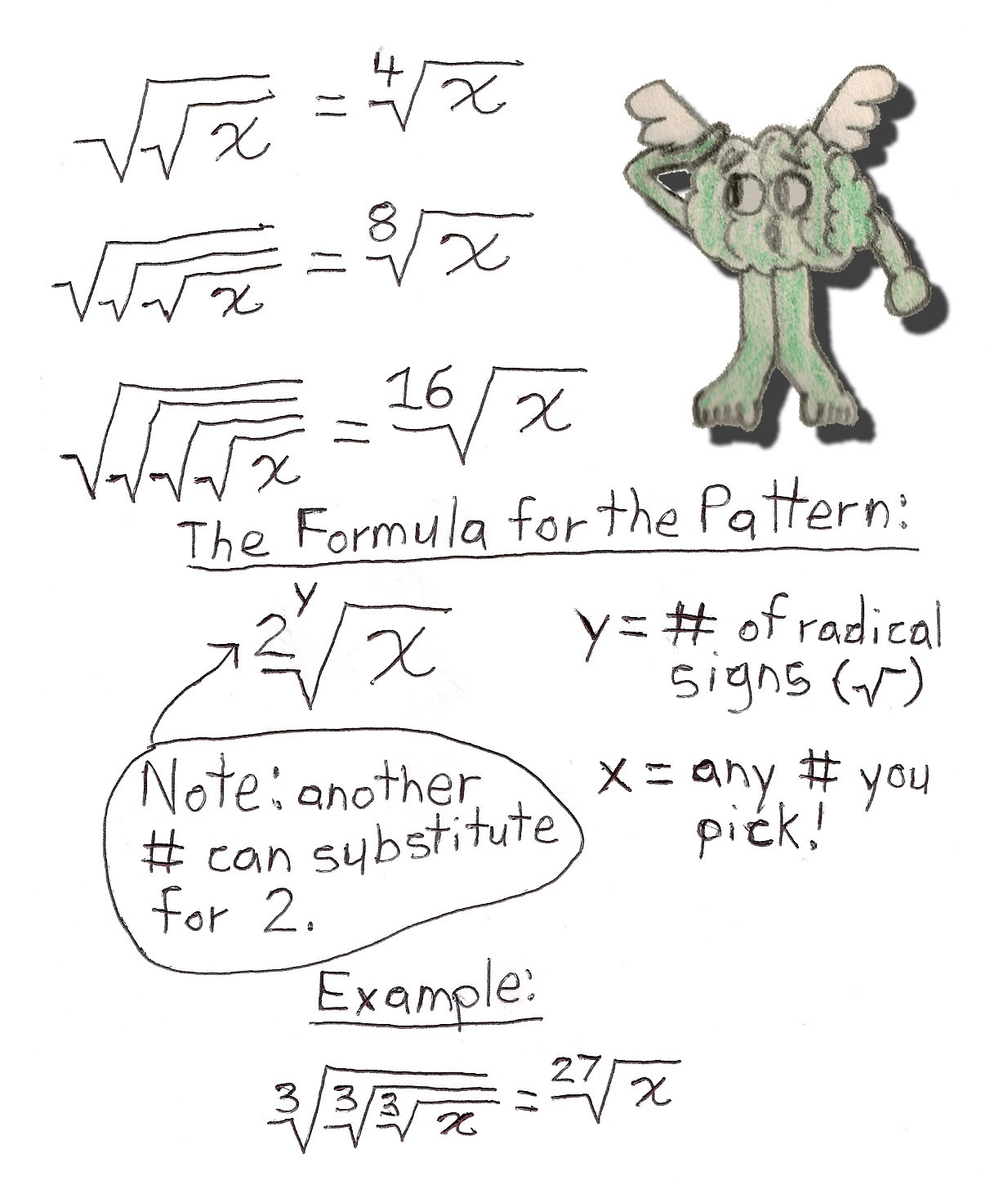# Root Repetition

## When you put radical signs over each other, something cool happens to the number underneath them! Observe below:The square root of a square root is the 4th root of a number, & the square root of that is the 8th root, & the square root of that is the 16th root, etc. As pictured above, another number can substitute for 2 on the radical signs, like 3 as in this example; the cube root of a cube root is the 9th root of a number, & the cube root of that is the 27th root, & the cube root of that is the 81st root, etc. It's exponential!